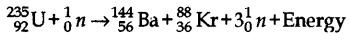## ICSE Physics Previous Year Question Paper 2019 Solved for Class 10

ICSE Class 10 Physics Board Question Paper 2019 Solved Pdf

General Instructions :

• Answers to this Paper must he written on the paper provided separately.
• You will not be allowed to write during the first 15 minutes.
• This time is to he spent in reading the question paper.
• The time given at the head of this Paper is the time allowed for writing the answers.
• Section I is compulsory. Attempt any four questions from Section II.
• The intended marks for questions or parts of questions are given in brackets [ ].

Section – I[40 Marks]
(Attempt all questions from this Section)

Question 1.
(a) The diagram below shows a claw hammer used to remove a nail:(i) To which class of lever does it belong ?
(ii) Give one more example of the same class of lever mentioned by you in (i) for which the mechanical advantage is greater than one.
(b) Two bodies A and B have masses in the ratio 5:1 and their kinetic energies are in the ratio 125:9. Find the ratio of their velocities. 
(c) (i) Name the physical quantity which is measured in calories
(ii) How is calorie related to the SI unit of that quantity? 
(d) (i) Define couple.
(ii) State the SI unit of moment of couple. 
(e) (i) Define critical angle.
(ii) State one important factor which affects the critical angle of a given medium. 
(a) (i) Class One.
(ii) Shears used for cutting the thin metal sheets.

(b) Given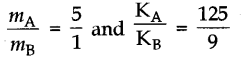Kinetic energy is given by K = $$\frac { 1 }{ 2 }$$ mv2
Therefore,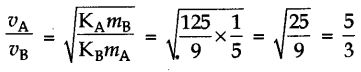(c) (i) Heat energy.
(ii) 1 cal = 4.2 J.

(d) (i) It is defined as a set of two equal and opposite forces acting along different lines
of action.
(ii) SI unit is N m.

(e) (i) It is the angle of incidence in the denser medium for which angle of refraction in the rarer medium is 90°.
(ii) Nature of the two media in contact.

Question 2.
(a) An electromagnetic radiation is used for photography in fog.
(ii) Why is this radiation mentioned by you, ideal for this purpose? 
(b) (b) (i) What is the relation between the refractive index of water with respect to air (aμw) and the refractive index of air with respect to water (wμa)
(ii) If the refractive index of water ivith respect to air (aμw) is $$\frac { 5 }{ 3 }$$. Calculate the refractive index of air ivith respect to water (wμa) 
(c) The specific heat capacity of a substance A is 3,800 J kg-1K-1 and that of a substance B is 400 J Kg-1K-1. Which of the two substances is a good conductor of heat? Give a reason for your answer. 
(d) A man playing a flute is able to produce notes of different frequencies. If he closes the holes near his mouth, will the pitch of the note produced, increase or decrease? Give a reason. 
(e) The diagram below shows a light source P embedded in a rectangular glass block ABCD of
critical angle 42°. Complete the path of the ray PQ till it emerges out of the block. [Write necessary angles.]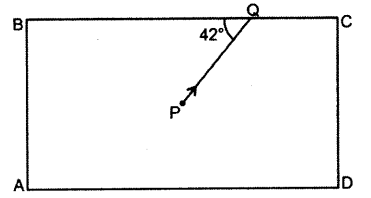(ii) Because it can penetrate fog.

(b)(ii) Given (aμw) = $$\frac { 5 }{ 3 }$$

Therefore, We have(c) Substance B. Because it requires relatively Httle heat to raise its temperature.

(d) The pitch will decrease, as the pitch is inversely proportional to the length of the vibrating air column.

(e) The path of ray is as shown: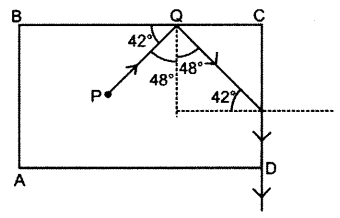Question 3.
(a) (i) If the lens is placed in water instead of air, how does its focal length change?
(ii) Which lens, thick or thin has greater focal length? 
(b) Two waves of the same pitch have amplitudes in the ratio 1 : 3.
What will be the ratio of their:
(i) intensities and
(ii) frequencies? 
(c) How does an increase in the temperature affect the specific resistance of a :
(i) Metal and
(ii) Semiconductor? 
(d) (i) Define resonant vibrations.
(ii) Which characteristic of sound, makes it possible to recognize a person by his voice without seeing him ? 
(e) Is it possible for a hydrogen (11H) nucleus to emit an alpha particle? Give a reason for your
(a) (i) Its focal length increases.
(ii) Thin lens.

(b) (i) Ratio of intensities will be 1: 9.
(ii) Ratio of frequencies will be 1:1.

(c) (i) Increases.
(ii) Decreases.

(d) (i) If a body is made to vibrate with its fundamental frequency by an external source, then the vibrations are called resonant vibrations.
(ii) Quality.

(e) No, as it does not possess a helium nucleus.

Question 4.
(a) Calculate the effective resistance across AB :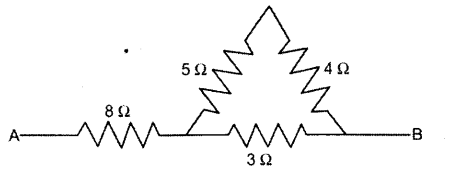(b) (i) State whether the specific heat capacity of a substance remains the same when its state changes from solid to liquid.
(c) A magnet kept at the centre of two coils A and B is moved to and fro as shouni in the diagram. The two galvanometers show deflection.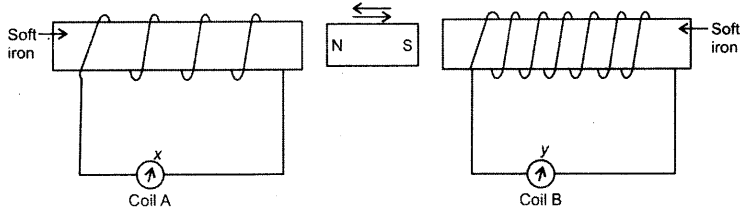State with a reason whether :
x > y or x <y [x and y are magnitudes of deflection] 
(d) (i) Why is a nuclear fusion reaction called a thermo nuclear reaction?
(ii) Complete the reaction : 
3 He2 + 2H14He2 +…….+Energy
(e) State two ways to increase the speed of rotation of a DC motor. 
(a) Resistors of 5 Ω and 4 Ω are in series, therefore, their net resistance is Rs = 5 + 4 = 9 Ω.
Now, this 9 Cl and 3 Ω are in parallel, hence net resistance RP = $$\frac{9 \times 3}{9+3}$$ = $$\frac { 18 }{ 12 }$$ = 1.5 Ω.
Now, this is in series with the 8 Cl resistor, hence net resistance of the circuit is RT = 8 + 1.5 = 9. 5

(b) (i) No, specific heat capacity does not remain the same when it changes from solid to liquid.
(ii) Let us take the example of water.
Specific heat capacity of water = 4.2 J kg-1 K-1
and specific heat capacity of ice = 2.1 J kg-1K-1

(c) x < y. This is because coil B has more number of turns.

(d) (i) Because this occurs at a very high temperature.
(ii) 3He2 + 2H14He2 + 1H1 + Energy

(e) The two ways are

1. Strong magnet and
2. High current.

Section – II [40 Marks]
(Attempt any four questions from this Section)

Question 5.
(a) A body of mass 10 kg is kept at a height of 5 m. It is allowed to fall and reach the ground.
(i) What is the total mechanical energy possessed by the body at the height of 2 m assuming it is a frictionless medium?
(ii) What is the kinetic energy possessed by the body just before hitting the ground? Take
g= 10 m s-2. 
(b) A uniform metre scale is in equilibrium as shown in the diagram :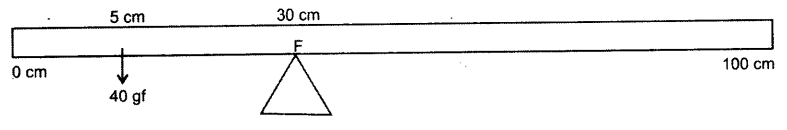(i) Calculate the weight of the metre scale.
(ii) Which of the following options is correct to keep the ruler in equilibrium when 40 gf wt is shifted to 0 cm mark?
F is shifted towards 0 cm or
F is shifted towards 100 cm. 
(c) The diagram below shows a pulley arrangement :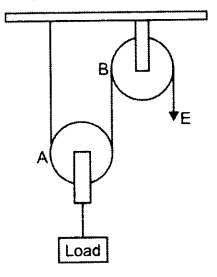(i) Copy the diagram and mark the direction of tension on each strand of the string.
(ii) What is the velocity ratio of the arrangement?
(iii) If the tension acting on the string is T, then what is the relationship between T and effort E?
(iv) If the free end of the string moves through a distance x, find the distance by which the
(a) (i) The total mechanical energy is the total potential energy it possesses before its fall.
E = mgh = 10 × 10 × 5 = 500 J
It is equal to the maximum potential energy E = mgh = 10 × 10 × 5 = 500 J.

(b) (i) The weight of the metre scale acts at its centre of gravity i.e., at 50 cm mark.
By the concept of moments, we have
40 × (30 – 5 ) = W × (50 – 30)
or W = (40 × 25)/ 20 = 50 gf
(ii) F is shifted towards 0 cm.

(c) (i) The direction of tension is as marked.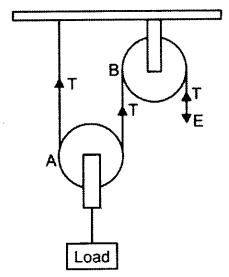(ii) VR = Distance travelled by effort/Distance travelled by load =$$\frac { 2x }{ 2 }$$ = 2.
The distance travelled by load is half the distance moved by effort = $$\frac { x }{ 2 }$$
(iii) T = E.
(iv) The distance travelled by load is half the distance moved by effort = $$\frac { x }{ 2 }$$ .

Question 6.
(a) How does the angle of deviation formed by a prism change with the increase in the angle of
incidence? Draw a graph showing the variation in the angle of deviation with the angle of incidence at a prism surface. 
(b) A virtual, diminished image is formed when an object is placed between the optical centre and the principal focus of a lens.
(i) Name the type of lens which forms the above image.
(ii) Draw a ray diagram to show the formation of the image with the above stated characteristics. 
(c) An object is placed at a distance 24 cm in front of a convex lens of focal length 8 cm.
(i) What is the nature of the image so formed ?
(ii) Calculate the distance of the image from the lens.
(iii) Calculate the magnification of the image. 
(a) As the. angle of incidence increases the angle of deviation decreases becomes minimum and then increases.
The graph is as shown :(b) (i) Concave lens.
(ii) The ray diagram is as shown :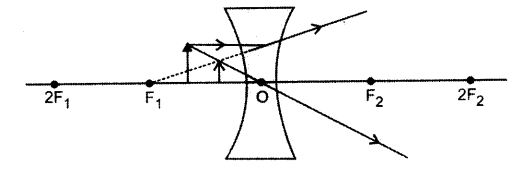(c) Given u = – 24 cm, f= + 8 cm, v = ?
(i) Real, inverted and diminished.
(ii) Using the lens formula(iii) Using the expression m =$$\frac { v }{ u }$$ = $$\frac { 12 }{ -24 }$$ = $$\frac { 1 }{ 2 }$$ .

Question 7.
(a) It is observed that during march-past we hear a base drum distinctly from a distance compared to the side drums.
(i) Name the characteristic of sound associated with the above observation.
(ii) Give a reason for the above observation. 
(b) A pendulum has a frequency of 4 vibrations per second. An observer starts the pendulum and fires a gun simultaneously. He hears the echo from the cliff after 6 vibrations of the pendulum. If the velocity of sound in air is 340 m s1, find the distance between the cliff and the observer. 
(c) Two pendulums C and D are suspended from a wire as shown in the given figure. Pendulum C is made to oscillate by displacing it from its mean position. It is seen that D also starts oscillating.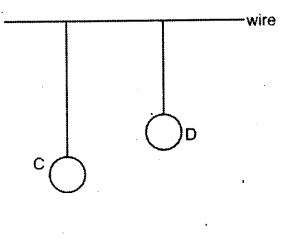(i) Name the type of oscillation, C will execute.
(ii) Name the type of oscillation, D will execute.
(iii) If the length of D is made equal to C, then what difference will you notice in the oscillations ofD?
(iv) WHat is the name of the phenomenon when the length of D is made equal to C? 
(a) (i) Intensity of sound.
(ii) This is because the amplitude of vibration of a base drum is far greater than the amplitude of vibration of the side drum.

(b) Given f= 4 vbs, T = $$\frac { 1 }{ f }$$ = $$\frac { 1 }{ 4 }$$ = 0.25 s, for a frequency of 6 vbs titme t= $$\frac { 0.25 }{ 4 }$$ x 6 = 0.375 s, ,V=340ms-1
Using S = $$\frac{\mathbf{V} \times t}{2}$$ = $$\frac{340 \times 0.375}{2}$$ = 63.75 m

(c) (i) Free or natural oscillation.
(ii) Forced oscillation.
(iii) The amplitude of the oscillation of D will be equal to the amplitude of the oscillation of C.
(iv) Resonance.

Question 8.
(a) (i) Write one advantage of connecting electrical appliances in parallel combination.
(ii) What characteristics should a fuse wire have?
(iii) Which wire in a power circuit is connected to the metallic body of the appliance? 
(b) The diagram below shows a dual control switch circuit connected to a bulb.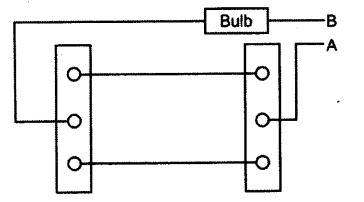(i) Copy the diagram and complete it so that the bulb is switched ON.
(ii) Out of A & B which one is the live wire and which one is the neutral wire?
(c) The diagram shows a circuit with the key k open.Calculate:
(i) the resistance of the circuit when the key k is crpen.
(ii) the current drawn from the cell when the key k is open,
(iii) the resistance of the circuit when the key k is closed.
(iv) the current drawn from thexell when the key k is closed.
(a) (i) All appliances will continue to work even if one of the appliance does not work.
(ii) High resistivity and low melting point.
(iii) The ground wire.

(b) (i) The diagram is as shown :(ii) A is connected to the live wire and B to neutral wire.

(c) (i) RS = 5 + 0.5 = 5.5 Ω
(ii) I=$$\frac { V }{ R }$$=$$\frac { 3.3 }{ 5.5 }$$= 0.6A
5×5
(iii) RP = $$\frac{5 \times 5}{5+5}$$ == 2.5 Ω, thus total resistance is RT = 2.5 +0.5 = 3.0

I= $$\frac{\mathrm{V}}{\mathrm{R}_{\mathrm{T}}}$$ = $$\frac { 3.3 }{ 3.0 }$$
= 1.1 A

Question 9.
(a) (i) Define Calorimetry.
(ii) Name the material used for making a Calorimeter.
(iii) Why is a Calorimeter made-up of thin sheets of the above material answered in (ii) ? 
(b) The melting point of naphthalene is 80°C and the room temperature is 30°C. A sample of
liquid naphthalene at 100°C is cooled down to the room temperature. Draw a temperature¬time graph to represent this cooling. In the graph, mark the region which corresponds to the freezing process. 
(c) 104 g of water at 30°C is taken in a calorimeter made of copper of mass 42 g. When a certain mass of ice at 0°C is added to it, the final steady temperature of the mixture after the ice has melted, was found to be 10°C. Find the mass of ice added.
[Specific heat capacity of water = 4.2 J g-1 °C-1  : Specific latent heat of fusion of ice – 336 J g-1 ; Specific heat capacity of copper = 0.4 J g-1 °C-1 ] 
(a) (i) Calorimetry is the measurement of heat,
(ii) Copper.
(iii) Copper has small specific heat capacity. The thin sheets ensure that the box has small heat capacity.

(b) The graph is as shown :(c) Mass of water m1 = 104 g
Temperature of water T1 = 30°C
Final temperature T2 = 10°C
Mass of calorimeter m2 = 42 g
Temperature of ice T3= 0°C
Let the mass of ice added be = x g
Total heat energy lost = (104 × 4.2 × 20)+(42 × 0.4 × 20) = 8736 + 336 = 9072 J
Total heat energy gained = (336 × x)+(x × 4.2 × 10) = 336x + 42x = 378x
By principle of calorimetry,
Heat lost = Heat gained
378x =9072
x = $$\frac { 9072 }{ 378 }$$ = 24g

Question 10.
(a) Draw a neat labelled diagram of an AC generator.
(b) (i) Define nuclear fission.
(ii) Rewrite and complete the following nuclear reaction by filling in the atomic number of Ba and mass number of Kr :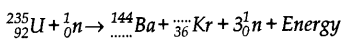(c) The diagram below shows a magnetic needle kept just below the conductor AB which is kept in North South direction.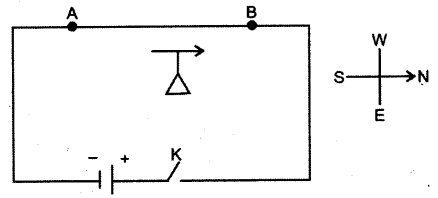(i) In which direction does the needle deflect when the key is closed ?
(ii) Why is the deflection produced?
(iii) What will he the change in the deflection if the magnetic needle is taken just above the conductor AB ?
(iv) Name one device which works on this principle.(ii)